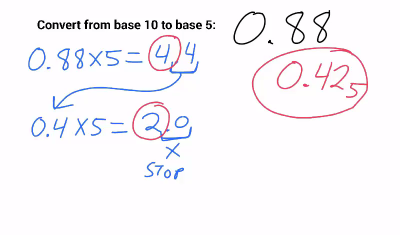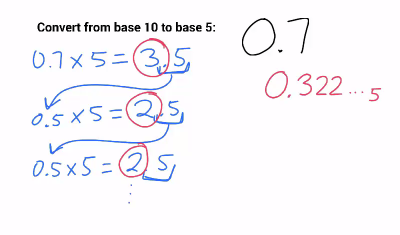# Chapter 10 Homework Help Videos

Here are links to a series of videos for Chapter 10 - Converting between different bases.

#### Video A - Converting Base 7 to Base 10 (1:57)

Converting whole numbers from base 7 to a base 10 (normal, decimal) number. Like Problem #1. (Three examples)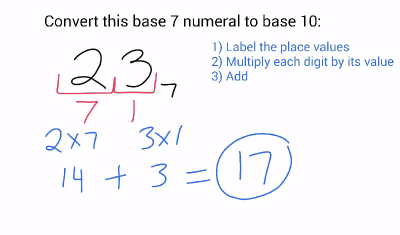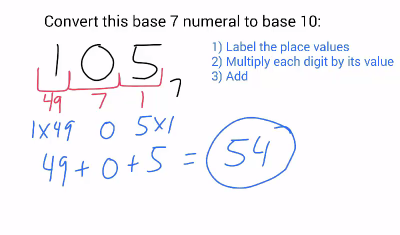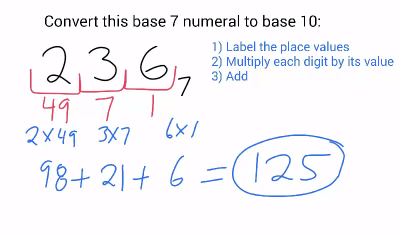#### Video B - Converting Base 10 to Base 7(1:37)

Converting whole numbers from base 10 to base 7. Like Problem #3. (Two examples)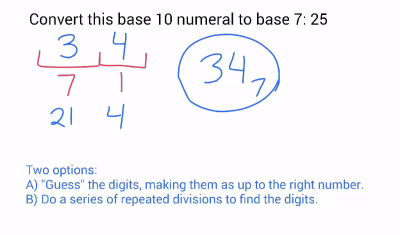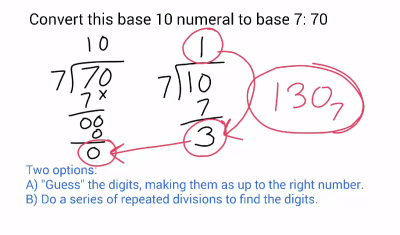#### Video C1 - Simple Addition (0:55)

A reminder of how to do long addition by hand. Nothing about different bases here, just an example of simple addition.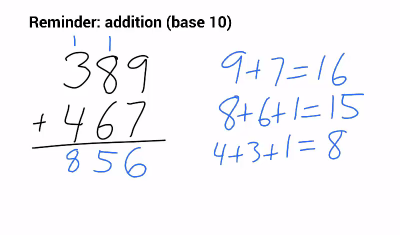#### Video C2 - Simple Multiplication (1:54)

A reminder of how to do long multiplication by hand. Nothing about different bases here, just an example of simple multiplication, in case you forgot how to do it. (You'll need to remember how to do this in normal numbers first, before you try to do it in different bases).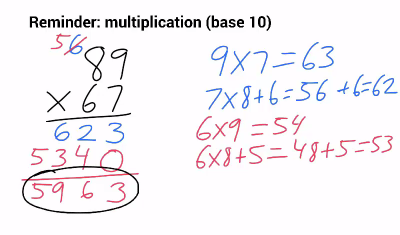#### Video D1 - Addition in Base 7 (1:24)

Adding two numbers in a different base. Like Problem #5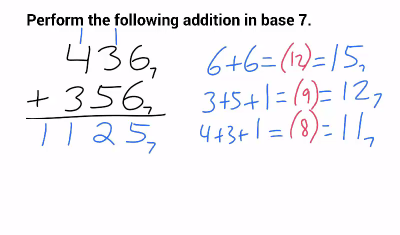#### Video D2 - Multiplication in Base 9 (2:25)

Multiplying two numbers in a different base. Like Problem #9.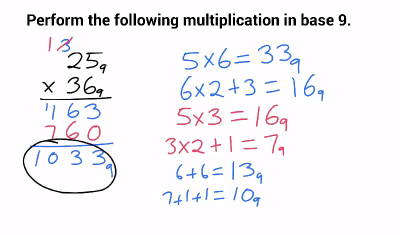#### Video E - Finding an unknown base(1:17)

Like problem #11. If a number is written in an unknown base, how do you find the base?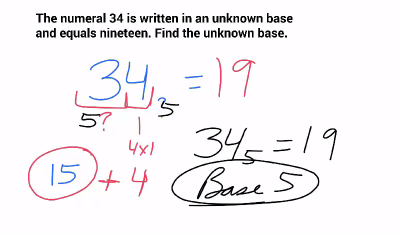#### Video F - Converting Decimals to Base 10 (1:21)

Convert from base 5 to base 10. Like problem #13.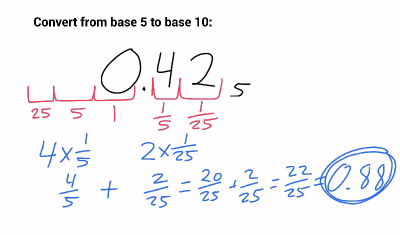#### Video G - Converting Decimals to Base 5 (2:21)

Convert from base 10 to base 5. Like problem #15. (Two examples)